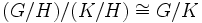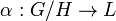# Endomorphism kernel is quotient-transitive

This article gives the statement, and possibly proof, of a subgroup property (i.e., endomorphism kernel) satisfying a subgroup metaproperty (i.e., quotient-transitive subgroup property)
View all subgroup metaproperty satisfactions | View all subgroup metaproperty dissatisfactions |Get help on looking up metaproperty (dis)satisfactions for subgroup properties
Get more facts about endomorphism kernel |Get facts that use property satisfaction of endomorphism kernel | Get facts that use property satisfaction of endomorphism kernel|Get more facts about quotient-transitive subgroup property

## Statement

Suppose$H \le K \le G$ are groups such that$H$ is an endomorphism kernel in$G$ and the quotient group$K/H$ is an endomorphism kernel in$G/H$. (Note that it makes sense to take quotients because endomorphism kernel implies normal). Then,$K$ is an endomorphism kernel in$G$.

## Definitions used

We use the following definition of endomorphism kernel: a normal subgroup$A$ of a group$B$ is an endomorphism kernel if there exists a subgroup of$B$ isomorphic to the quotient group$B/A$.

## Facts used

1. Third isomorphism theorem: This basically tells us that$(G/H)/(K/H) \cong G/K$.

## Proof

Given: Groups$H \le K \le G$ such that$H$ is an endomorphism kernel in$G$ and$K/H$ is an endomorphism kernel in$G/H$.

To prove:$K$ is an endomorphism kernel in$G$.

Proof:

Step no. Assertion/construction Facts used Given data used Previous steps used Explanation
1 Let$L$ be a subgroup of$G$ isomorphic to$G/H$ and let$\alpha:G/H \to L$ be an isomorphism between them.$H$ is an endomorphism kernel in$G$ Definition-direct
2 Let$M$ be a subgroup of$G/H$ isomorphic to$(G/H)/(K/H)$.$K/H$ is an endomorphism kernel in$G/H$. Definition-direct
3$(G/H)/(K/H)$ is isomorphic to$G/K$. Fact (1)$H \le K \le G$,$H$ normal in$G$,$K/H$ normal in$G/H$ (normality follows from being endomorphism kernels) Fact-direct
4$M$ is a subgroup of$G/H$ isomorphic to$G/K$. Steps (2), (3) step-combination direct
5$\alpha(M)$ is a subgroup of$L$ (and hence of$G$) isomorphic to$M$ Steps (1), (2)$\alpha:G/H \to L$ is an isomorphism, hence it maps subgroups to subgroups isomorphic to them. Apply this to$M$.
6$\alpha(M)$ is a subgroup of$G$ isomorphic to$G/K$. Steps (4), (5) step-combination direct.
7$K$ is an endomorphism kernel in$G$. Step (6) definition-direct.